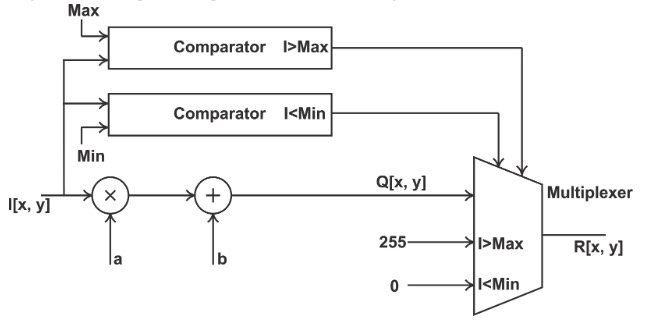Technical Article

# How to Conduct Point Operations for Digital Image Processing: Using Look-up Tables

November 03, 2019 by Dr. Steve Arar

## Learn how to use look-up tables to conduct point operations in digital image processing.

In the last article, we discussed point operations used in digital image processing. Here, we will go through how to implement these operations using a look-up table-based methodology on an example image.### The Implementation of Point Operations

Assume that we have an 8-bit grayscale image of size 512512pixels. We need to apply the following transfer function to adjust the image brightness and contrast:

Q [x, y] = a x I [x, y] + b

where a and b are assumed to be constant known values. Suppose that we want to have an implementation that saturates the output of the above transfer function when it exceeds the range 0 to 255.

One possible implementation is shown below.##### Figure 2

Since we have assumed that a and b are known and constant, we can determine threshold pixel values that will be mapped to a value outside the range 0 to 255. These upper and lower limits are denoted by “Max” and “Min” in the above figure. An input pixel value is compared with these threshold values and depending on the result of these comparisons Q[x, y], 255, or 0 is passed on to the multiplexer output. How many operations are required to process the whole image? There are 512 x 512 = 218 pixels to process.

For each pixel, we should do one multiplication, one addition, and two digital comparisons (We are ignoring the multiplexing operation). Hence, a total of 4 x 218 = 220 operations are required!

Is there a more efficient implementation that hopefully leads to a smaller circuit and/or power consumption?

### Look-up Table-Based Implementation

In addition to using logic gates to implement the transfer function of a given point operation, we can also consider a look-up table-based implementation.

The look-up table based implementation becomes particularly more efficient when several point operations are cascaded. To better understand why a look-up table can be an efficient alternative to implementing the logic itself, we should note that there might be several repeated operations when processing the pixels of an image only based on the pixel value.

In the above example, we have 512 x 512 = 218 pixels to process.

In an eight-bit grayscale image, a pixel can take only 28 different values. Considering the total number of pixels (218) and the different values that a pixel can have (28), we can conclude that there is at least one pixel value that appears at least $$\frac {2^{18}}{2^8}=2^{10}$$ =1024 times in the matrix of our grayscale image.

The logic implementation of the figure above processes all of the pixels in an independent way and does not consider that many of these computations are repeated. However, noting the fact that each pixel can be a value with only 28 different levels, we can use a look-up table to store the output of our transfer function for each of these pixel values.

Hence, our eight-bit pixel value will be connected to the 8-bit address of this look-up table. Since the output of the transfer function is an eight-bit value, we need a 256 x 8-bit look-up table to implement this example.

### The Benefits of Using the Look-up Table Method

The look-up table based method can lead to a more efficient solution particularly if several different point operations are cascaded. This is due to the fact that with a look-up table, we can pre-calculate the final output of all of these cascaded transfer functions and store these final values in the look-up table.

Note that the look-up table-based method can be used only if the design is not parameterized and we are able to precalculate the output for a given input pixel value. However, if there are only a small number of parameters, we might still be able to use a combination of some logic gates along with a few look-up tables to have a more efficient implementation.

This concludes the example portion of learning how to conduct point operations in digital image processing. If you have questions about the information herein, please leave a comment below.